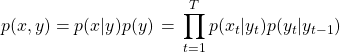## What is the complexity of Viterbi algorithm ?

Viterbi algorithm is a dynamic programming approach to find the most probable sequence of hidden states given the observed data, as modeled  by a HMM.  Without dynamic programming, it becomes an exponential problem as there are exponential number of possible sequences for a given observation(How – explained in answer below). Let the transition probabilities(state transition)…

## How do you find the most probable sequence of POS tags from a sequence of text?

This problem can be solved with a HMM. Using a HMM involves finding the transition probabilities (what is the probability of going from one POS tag to another and emission/output probabilities (what is the probability of observing a word given a POS tag) as explained in the question How do you train an hMM. Once…

## How do you train a hMM model in practice ?

The joint probability distribution for the HMM model is given by the following equation where are the observed data points and the corresponding latent states:     Before proceeding to answer the question on training a HMM, it makes sense to ask following questions What is the problem in hand for which we are training…

## What are the different independence assumptions in hMM & Naive Bayes ?

Both the hMM and Naive Bayes have conditional independence assumption. hMM can be expressed by the equation below :         Second equation implies a conditional independence assumption: Given the state observed variable is conditionally independent of previous observed variables, i.e. and Naive Bayes Model is expressed as:     is the feature…

## How do you generate text using a hMM model given below:One possible interpretation of the latent variables in the HMM model is that they are POS tags. We will go with this interpretation for simplicity, though the latent states could mean other things as well. To generate text from a HMM, we need to know the transition matrix (the probability of going from one tag…

## What order of Markov assumption does n-grams model make ?

An n-grams model makes order n-1 Markov assumption. This assumption implies: given the previous n-1 words, probability of  word is independent of words prior to words. Suppose we have k words in a sentence, their joint probability can be expressed as follows using chain rule:      Now, the Markov assumption can be used to make…

## How is long term dependency maintained while building a language model?

Language models can be built using the following popular methods – Using n-gram language model n-gram language models make assumption for the value of n. Larger the value of n, longer the dependency. One can refer to what is the significance of n-grams in a language model for further reading. Using hidden Markov Model(HMM) HMM maintains long…